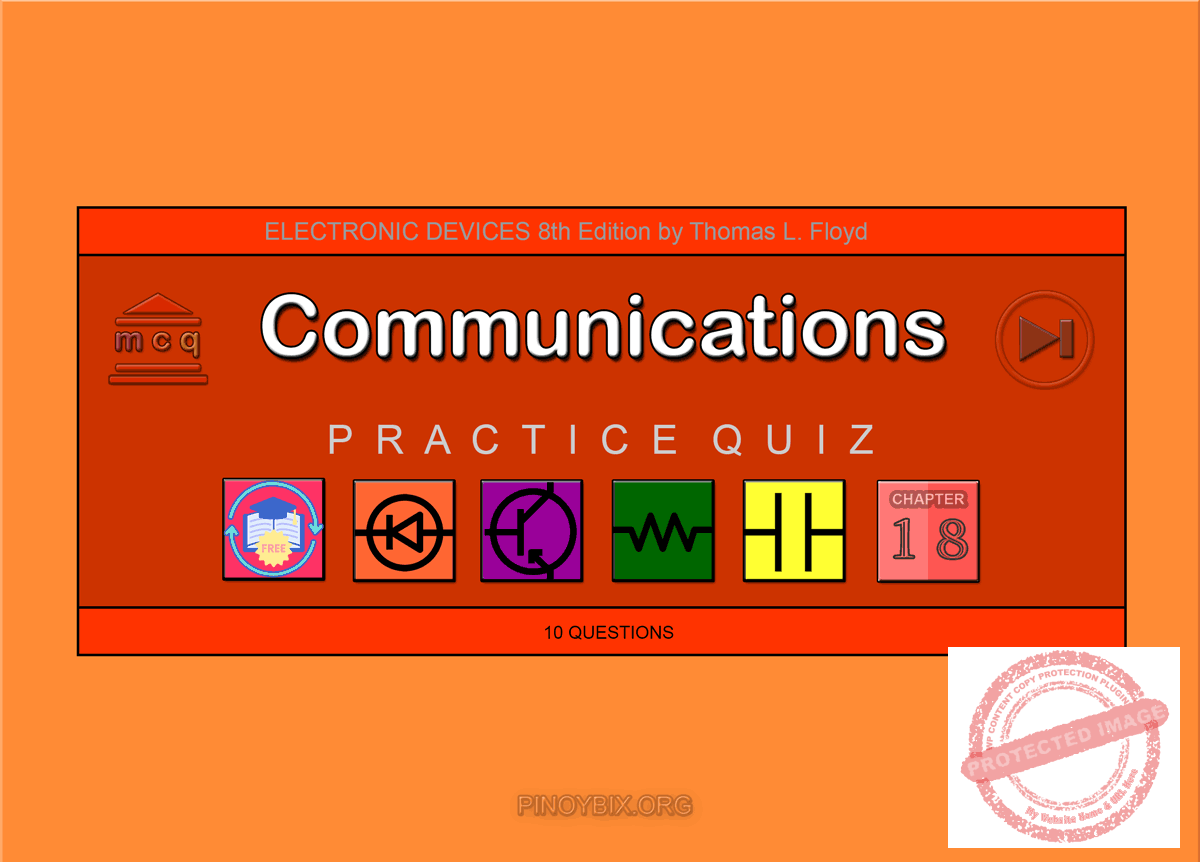# Floyd: MCQ in Communications

(Last Updated On: December 2, 2019)This is the Multiple Choice Questions in Communications from the book Electronic Devices – Electron Flow Version and Conventional Current Version 8th Edition by Thomas L. Floyd. If you are looking for a reviewer in Electronics Engineering this will definitely help. I can assure you that this will be a great help in reviewing the book in preparation for your Board Exam. Make sure to familiarize each and every questions to increase the chance of passing the ECE Board Exam.

#### Practice Exam Test Questions

Choose the letter of the best answer in each questions.

1. In AM, the carrier is changed by a modulating signal. What parameter of the carrier is changed?

A. amplitude

B. frequency

C. pulse width

D. phase

Solution:

2. The intermediate frequency in a standard AM receiver is

A. 455 Hz.

B. 455 kHz.

C. 4.55 MHz.

D. none of the above

Solution:

3. If a 1 MHz carrier is amplitude modulated with a 5 kHz audio signal, the upper-side frequency is _____ kHz.

A. 1005

B. 1000

C. 995

D. none of the above

Solution:

4. For a four-quadrant linear multiplier, what is the output voltage, given input voltages of Vx = +2 V and Vy = –10 V?

A. +2 V

B. +1 V

C. –2 V

D. –1 V

Solution:

5. Amplitude modulation is a _____ process.

A. multiplication

B. division

C. sum/difference

Solution:

6. The output spectrum of a _____ modulator includes upper-side and lower-side frequencies and the carrier frequency.

A. balanced

B. standard amplitude

C. none of the above

D. none of the above

Solution:

7. An AM demodulator can be implemented with a linear multiplier followed by a _____ filter.

A. low-pass

B. high-pass

C. band-pass

D. band-stop

Solution:

8. What does VCO stand for?

A. Visually-Controlled Organization

B. Voltage-Controlled Oscillator

C. Voltage-Centered Oscilloscope

D. none of the above

Solution:

9. A phase-locked loop (PLL) is a feedback circuit consisting of a

A. phase detector.

B. low-pass filter.

C. VCO.

D. all of the above

Solution:

10. Light may be propagated along a fiber-optic cable in which of the following modes?

A. multimode step index

B. single-mode step index

D. all of the above

Solution:

11. In order for a light ray to propagate along a fiber-optic cable, what must the relationship be between the angle of incidence and the critical angle?

A. θi > θc

B. θi < θc

C. θi = θc

D. none of the above

Solution:

12. A certain fiber-optic cable has the following characteristics: n1 = 1.82 and n2 = 1.73. What is the value of θc?

A. 1.0°

B. 0.95°

C. 18.1°

D. 1.81°

Solution:

#### TRUE/FALSE

1. Amplitude modulation is the process of varying the radio frequency with the modulating frequency.

A. True

B. False

Solution:

2. The purpose of the balanced modulator is to remove the carrier frequency.

A. True

B. False

Solution:

3. An AM signal is made up of a carrier, an upper-side frequency, and a lower-side frequency.

A. True

B. False

Solution:

4. The mixer takes the RF signal along with the audio signal to produce the IF signal.

A. True

B. False

Solution:

5. Selectivity in a receiver is provided by the resonant circuits of the IF section.

A. True

B. False

Solution:

6. Frequency modulation is achieved by varying the frequency of an oscillator with a modulating signal.

A. True

B. False

Solution:

7. A VCO is a variable capacitance oscillator.

A. True

B. False

Solution:

8. The demodulator recovers the audio signal from the IF output.

A. True

B. False

Solution:

9. The IF of an FM receiver operates at a center frequency of 455 kHz.

A. True

B. False

Solution:

10. A balanced modulator is used in standard AM to obtain the sum and difference frequencies.

A. True

B. False

Solution:

PinoyBIX Engineering. © 2014-2021 All Rights Reserved | How to Donate? |